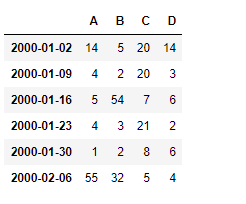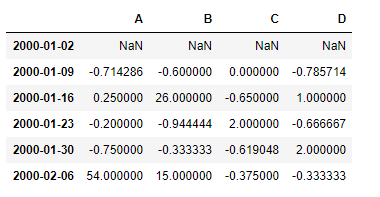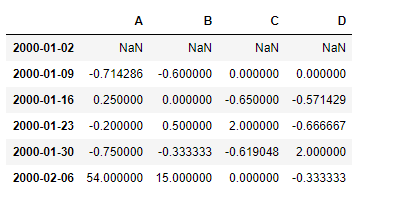GFG App
Open AppBrowser
Continue

# Python | Pandas dataframe.pct_change()

Python is a great language for doing data analysis, primarily because of the fantastic ecosystem of data-centric python packages. Pandas is one of those packages and makes importing and analyzing data much easier.

Pandas` dataframe.pct_change()` function calculates the percentage change between the current and a prior element. This function by default calculates the percentage change from the immediately previous row.

Note : This function is mostly useful in the time-series data.

Syntax: DataFrame.pct_change(periods=1, fill_method=’pad’, limit=None, freq=None, **kwargs)

Parameters :
periods : Periods to shift for forming percent change.
fill_method : How to handle NAs before computing percent changes.
limit : The number of consecutive NAs to fill before stopping
freq : Increment to use from time series API (e.g. ‘M’ or BDay()).
**kwargs : Additional keyword arguments are passed into DataFrame.shift or Series.shift.

Returns : The same type as the calling object.

Example #1: Use `pct_change()` function to find the percentage change in the time-series data.

 `# importing pandas as pd ` `import` `pandas as pd ` ` `  `# Creating the time-series index ` `ind ``=` `pd.date_range(``'01/01/2000'``, periods ``=` `6``, freq ``=``'W'``) ` ` `  `# Creating the dataframe  ` `df ``=` `pd.DataFrame({``"A"``:[``14``, ``4``, ``5``, ``4``, ``1``, ``55``], ` `                   ``"B"``:[``5``, ``2``, ``54``, ``3``, ``2``, ``32``],  ` `                   ``"C"``:[``20``, ``20``, ``7``, ``21``, ``8``, ``5``], ` `                   ``"D"``:[``14``, ``3``, ``6``, ``2``, ``6``, ``4``]}, index ``=` `ind) ` ` `  `# Print the dataframe ` `df `Let’s use the `dataframe.pct_change()` function to find the percent change in the data.

 `# find the percentage change with the previous row ` `df.pct_change() `

Output :The first row contains `NaN` values, as there is no previous row from which we can calculate the change.

Example #2: Use `pct_change()` function to find the percentage change in the data which is also having `NaN` values.

 `# importing pandas as pd ` `import` `pandas as pd ` ` `  `# Creating the time-series index ` `ind ``=` `pd.date_range(``'01/01/2000'``, periods ``=` `6``, freq ``=``'W'``) ` ` `  `# Creating the dataframe  ` `df ``=` `pd.DataFrame({``"A"``:[``14``, ``4``, ``5``, ``4``, ``1``, ``55``], ` `                   ``"B"``:[``5``, ``2``, ``None``, ``3``, ``2``, ``32``],  ` `                   ``"C"``:[``20``, ``20``, ``7``, ``21``, ``8``, ``None``], ` `                   ``"D"``:[``14``, ``None``, ``6``, ``2``, ``6``, ``4``]}, index ``=` `ind) ` ` `  `# apply the pct_change() method ` `# we use the forward fill method to ` `# fill the missing values in the dataframe ` `df.pct_change(fill_method ``=``'ffill'``) `

Output :The first row contains `NaN` values, as there is no previous row from which we can calculate the change. All the `NaN` values in the dataframe has been filled using `ffill` method.

My Personal Notes arrow_drop_up